Courses

# Conservation of Mechanical Energy & Various Forms of Energy JEE Notes | EduRev

## JEE : Conservation of Mechanical Energy & Various Forms of Energy JEE Notes | EduRev

The document Conservation of Mechanical Energy & Various Forms of Energy JEE Notes | EduRev is a part of the JEE Course Physics Class 11.
All you need of JEE at this link: JEE

Conservation of Mechanical Energy

Have you ever wondered how an automatic mechanical watch works? At the center of its complicated motion lies one of the most basic principles of classical physics: The law of conservation of mechanical energy. Let’s delve into the principle:

Mechanical Energy

It is the capacity of an object to do work by the virtue of its motion or configuration (position). Mechanical Energy is the sum of following two energy terms:

• Kinetic Energy. It is the ability of an object to do work by the virtue of its motion. For example, the kinetic energy of Wind has the capacity to rotate the blades of a windmill and hence produce electricity. Kinetic energy is expressed as,where, K is the kinetic energy of the object in joules (J), m is the mass of the object in kilograms and v is the velocity of the object: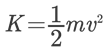• Potential energy. It is the ability of an object to do work by the virtue of its configuration or position. For example, a compressed spring can do work when released. For the purpose of this article, we will focus on the potential energy of an object by the virtue of its position with respect to the earth’s gravity. Potential energy can be expressed as:
V = mg h

Here, V is the potential energy of the object in joules (J), m is the mass of the object in kilograms, g is the gravitational constant of the earth (9.8 m/s2), and h is the height of the object from earth’s surface. Now, we know that the acceleration of an object under the influence of earth’s gravitational force will vary according to its distance from the earth’s centre of gravity.
But, the surface heights are so minuscule when compared to the earth’s radius, that, for all practical purposes, g is taken to be a constant.

Conservation of Mechanical Energy

The sum total of an object’s kinetic and potential energy at any given point in time is its total mechanical energy. The law of conservation of energy says “Energy can neither be created nor be destroyed.”
So, it means, that, under a conservative force, the sum total of an object’s kinetic and potential energies remains constant.  Before we dwell on this subject further, let us concentrate on the nature of a conservative force.

Conservative Force

A force is said to be conservative if work done by or against the force in moving a body depends only on the initial and final positions of the body and does not depend on the nature of path followed between the initial and final positions.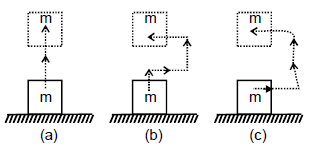Consider a body of mass m being raised to a height h vertically upwards as shown in above figure. The work done is mgh. Suppose we take the body along the path as in (b). The work done during horizontal motion is zero. Adding up the works done in the two vertical parts of the paths, we get the result mgh once again. Any arbitrary path like the one shown in (c) can be broken into elementary horizontal and vertical portions. Work done along the horizontal path is zero. The work done along the vertical parts add up to mgh. Thus we conclude that the work done in raising a body against gravity is independent of the path taken. It only depends upon the initial and final positions of the body. We conclude from this discussion that the force of gravity is a conservative force.

Properties of Conservative forces

• Work done by or against a conservative force depends only on the initial and final position of the body.
• Work done by or against a conservative force does not depend upon the nature of the path between initial and final position of the body.
• Work done by or against a conservative force in a round trip is zero.
• If a body moves under the action of a force that does no total work during any round trip, then the force is conservative; otherwise it is non-conservative.
• The concept of potential energy exists only in the case of conservative forces.
• The work done by a conservative force is completely recoverable.

Examples of Conservative forces.

(i) Gravitational force, not only due to Earth due in its general form as given by the universal law of gravitation, is a conservative force.

(ii) Elastic force in a stretched or compressed spring is a conservative force.

(iii) Electrostatic force between two electric charges is a conservative force.

(iv) Magnetic force between two magnetic poles is a conservative force.

Forces acting along the line joining the centers of two bodies are called central forces. Gravitational force and Electrostatic forces are two important examples of central forces. Central forces are conservative forces.

Non-conservative forces :

A force is said to be non-conservative if work done by or against the force in moving a body depends upon the path between the initial and final positions.

The frictional forces are non-conservative forces. This is because the work done against friction depends on the length of the path along which a body is moved. It does not depend only on the initial and final positions. Note that the work done by frictional force in a round trip is not zero.

The velocity-dependent forces such as air resistance, viscous force, magnetic force etc., are non conservative forces.

Difference between conservative and Non-conservative forces

 s.No. Conservative forces Non-Conservative forces 1 Work done does not depend upon path Work done depends on path. 2 Work done in a round trip is zero. Work done in a round trip is not zero. 3 Central in nature. Forces are velocity- dependent and retarding in nature. 4 W'hen            only             aconservative force acts within a system, the kinetic energy and potential energy can change. However their sum, the mechanical energy of the system, does not change. Work done against a non conservative force may be dissipated as heat energy. 5 Work            done            iscompletely recoverable. Work done in not completely recoverable.

Proof of Conservation of Mechanical energy

Let us consider the following illustration: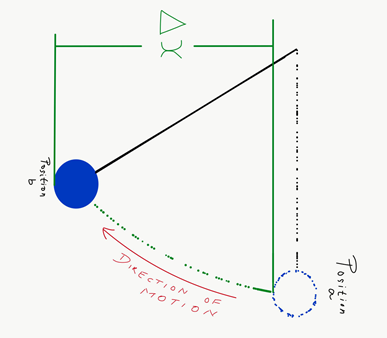Here, Δx is the displacement of the object under the conservative force F. By applying the work-energy theorem, we have: ΔK = F(x) Δx. Since the force is conservative, the change in potential Energy can be defined as ΔV = – F(x) Δx. Hence,

ΔK + ΔV = 0 or Δ(K + V) = 0

Therefore for every displacement of Δx, the difference between the sums of an object’s kinetic and potential energy is zero. In other words, the sum of an object’s kinetic and potential energies is constant under a conservative force. Hence, the conservation of mechanical energy is proved.

Case Study: Simple Pendulum

The pendulum is a very good example of conservation of mechanical energy.  Following illustration will help us understand the pendulum motion: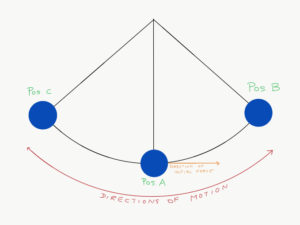• At position A, Potential energy is zero and the kinetic energy is at maximum.
• When the object travels from position A to B, it’s kinetic energy reduces and potential energy increases.
• At position B, the object stops momentarily.  At this position, the object’s kinetic energy becomes zero and its potential energy reaches the maximum.  The law of conservation of mechanical energy comes into play here.  The object’s entire kinetic energy at position A has been converted to potential energy at position B.
• Now, the object retraces its path, this time from position B to position A.  Back at position A, the object’s kinetic energy has been restored to its initial level.  Object’s Potential energy is zero.
• Now, the object travels the exact same path as AB, but in reverse direction of AC.
• This process repeats itself infinitely because the mechanical energy of the object remains constant.

This property of mechanical energy has been harnessed by watchmakers for centuries. Of course, in the real world, one has to account the other forces like friction and electromagnetic fields. Hence, no mechanical watch can run perpetually. But, if you get a precise mechanical watch like Rolex, you can expect long power reserves!

Solved Examples For You

Q: A mass of 2kg is suspended by a light string of length 10m. It is imparted a horizontal velocity of 50m/s. Calculate the speed of the said mass at point B.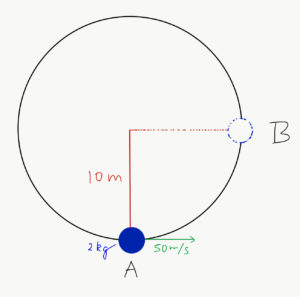Solution: Potential energy at point A, V(A) = mgh(A)
Kinetic energy at point A, K(A) = (mv²)/2 = (2 × 2500)/2 = 2500J
Hence, total mechanical energy at point A, K(A) + V(A) = [2500 + V(A)]J
Potential energy at point B, V(B) = mg h(B) = mgh (A+10) = mg h(A) + 2 × 9.8 × 10
= [V(A) + 196]J
Kinetic energy at point B, K(B) = (mv2)/2
Hence, total mechanical energy at point B, K(B) + V(B) = [K(B) + V(A) + 196]J
By applying the law of conservation of energy,
V(A) + K(A) = V(B) + K(B)
Therefore, V(A) + 2500 = K(B) + V(A) + 196
or K(B) = 2500 – 196
Which gives: (mv2)/2 = 2304
(2 × v2)/2 = 2304
v = ½
Therefore, velocity of the mass at point B = 48m/s

Work done by conservative forces

Ist format : (When constant force is given)

Ex.8 Calculate the work done to displace the particle from (1, 2) to (4, 5). if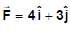Sol.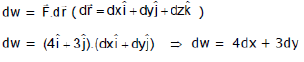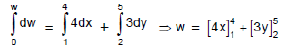w = (16 - 4) + (15 - 6) = w = 12 + 9 = 21 Joule

II format : (When F is given as a function of x, y, z)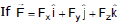then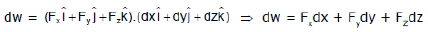Ex.9 An object is displaced from position vector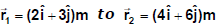under a force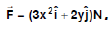Find the work done by this force.

Sol.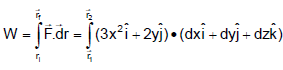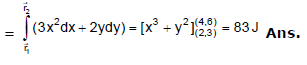IIIrd format (perfect differential format)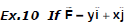then find out the work done in moving the particle from position (2, 3) to (5, 6)

Sol.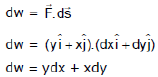Now ydx + xdy = d(xy) (perfect differential equation)
⇒ dw = d(xy)
for total work done we integrate both side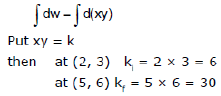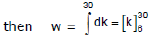⇒ w = (30 - 6) = 24 Joule

Internal Work

Suppose that a man sets himself in motion backward by pushing against a wall. The forces acting on the man are his weight 'W' the upward force N exerted by the ground and the horizontal force N exerted by the wall. The works of 'W' and of N are zero because they are perpendicular to the motion. The force N' is the unbalanced horizontal force that imparts to the system a horizontal acceleration. The work of N', however, is zero because there is no motion of its point of application. We are therefore confronted with a curious situation in which a force is responsible for acceleration, but its work, being zero, is not equal to the increase in kinetic energy of the system.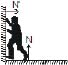The new feature in this situation is that the man is a composite system with several parts that can move in relation to each other and thus can do work on each other, even in the absence of any interaction with externally applied forces. Such work is called internal work. Although internal forces play no role in acceleration of the composite system, their points of application can move so that work is done; thus the man's kinetic energy can change even though the external forces do no work.

"Basic concept of work lies in following lines

Draw the force at proper point where it acts that give proper importance of the point of application of force.

Think independently for displacement of point of application of force, Instead of relation the displacement of applicant point with force relate it with the observer or reference frame in which work is calculated.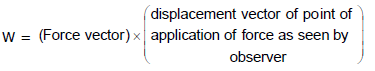Ex.11 Calculate the work done by the force to move the particle from (0, 0) to (1, 1) in the following condition

(a) y = x (b) y = x2

Sol. We know that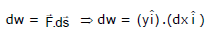dw = ydx ...(1)

In equation (1) we can calculate work done only when we know the path taken by the particle.

either

y = x or y = x2 so now

(a) when y = x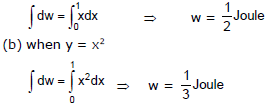Law of Conservation of Energy

Energy can be classified into two distinct categories : one based on motion, namely kinetic energy; the other onconfiguration (position), namely potential energy. Energy comes in many a forms which transform into one another in ways which may not often be clear to us.

The law of conservation of energy states that the total energy is constant in any process. Energy may change in form or be transferred from one system to another, but the total remains the same.

Different Forms of Energy

What is Energy?

We can define energy as the capacity to do work. There are various forms of energy. The law of conservation of energy states that energy can neither be created nor be destroyed. It can only be transferred from one form to another. Some forms of energy are defined and discussed in this article.Heat Energy

When you leave a cup of hot coffee unattended for some time, you find that the coffee has cooled down. This is because it lost heat to the surrounding which is at a lower temperature when compared to the coffee. Heat energy is the form of energy that results from the total kinetic energy of the particles in any object.
It is transferred as a result of a random motion of molecules which leads to them bouncing off each other and transferring energy in the process. It is also sometimes referred to as the energy in transit. Heat flows from a body of higher temperature (high total kinetic energy of the particles) to a body of lower temperature (low total kinetic energy of the particles).

Chemical Energy

Plants use solar energy, carbon dioxide, and water to synthesize food. This food is consumed by humans and animals, is broken down in their bodies, and they get energy for life activities. They are all examples of chemical energy.
Chemical energy is the energy that is stored in the bonds of chemical compounds. Moreover, a chemically stable compound has less energy stored in it as compared to its separate parts. When a chemical reaction takes place, there is a change in the arrangement of atoms which results in either absorption (endothermic) or a release (exothermic) of energy.

Electrical Energy

The energy due to the moving electrical charges is called electrical energy. So when you turn on a torch, the chemical energy stored in batteries makes the charged particles flow from the low potential terminal to a high potential terminal. This electrical energy lights the torch which is transferred to the surrounding as light energy.

Nuclear Energy

You might be aware of the infamous bombings of the cities of Hiroshima and Nagasaki during World War II which caused the destruction to the extent that its consequences can be seen even today. Those were the nuclear bombs which are considered as one of the most dangerous inventions of mankind till date. Nuclear energy is the energy in the nucleus of an atom. The energy is released during nuclear fusion or fission reactions. And the energy released is really vast. When the reaction is controlled, it can be used to generate electricity. Under uncontrolled nuclear fission reactions, nuclear weapons are created.

The Equivalence of Mass and Energy

An equation given by Einstein explains the relationship equivalence of mass and energy. According to it, the energy of a body is equal to the products of the mass of the body and the square of the speed of light. The famous equation is

E = mc2

Where E is the energy, m is the mass of the body and c is the speed of light in a vacuum which is approximately equal to 3 x 108 m/sec.

Offer running on EduRev: Apply code STAYHOME200 to get INR 200 off on our premium plan EduRev Infinity!

## Physics Class 11

75 videos|312 docs|152 tests

,

,

,

,

,

,

,

,

,

,

,

,

,

,

,

,

,

,

,

,

,

;